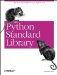# The getopt Module

The getopt module used in Example 2-23 contains functions to extract command-line options and arguments. It can handle both short and long option formats.

The second argument specifies the short options that should be allowed. A colon (:) after an option name means that option must have an additional argument.

Example 2-23. Using the getopt Module

```File: getopt-example-1.py

import getopt
import sys

# simulate command-line invocation
sys.argv = ["myscript.py", "-l", "-d", "directory", "filename"]

# process options
opts, args = getopt.getopt(sys.argv[1:], "ld:")

long = 0
directory = None

for o, v in opts:
if o == "-l":
long = 1
elif o == "-d":
directory = v

print "long", "=", long
print "directory", "=", directory
print "arguments", "=", args

long = 1
directory = directory
arguments = ['filename']```

To make getopt look for long options, as in Example 2-24, pass a list of option descriptors as the third argument. If an option name ends with an equals sign (=), that option must have an additional argument.

Example 2-24. Using the getopt Module to Handle Long Options

```File: getopt-example-2.py

import getopt
import sys

# simulate command-line invocation
sys.argv = ["myscript.py", "--echo", "--printer", "lp01", "message"]

opts, args = getopt.getopt(sys.argv[1:], "ep:", ["echo", "printer="])

# process options
echo = 0
printer = None

for o, v in opts:
if o in ("-e", "--echo"):
echo = 1
elif o in ("-p", "--printer"):
printer = v

print "echo", "=", echo
print "printer", "=", printer
print "arguments", "=", args

echo = 1
printer = lp01
arguments = ['message']```Python Standard Library (Nutshell Handbooks) with
ISBN: 0596000960
EAN: 2147483647
Year: 2000
Pages: 252
Authors: Fredrik Lundh